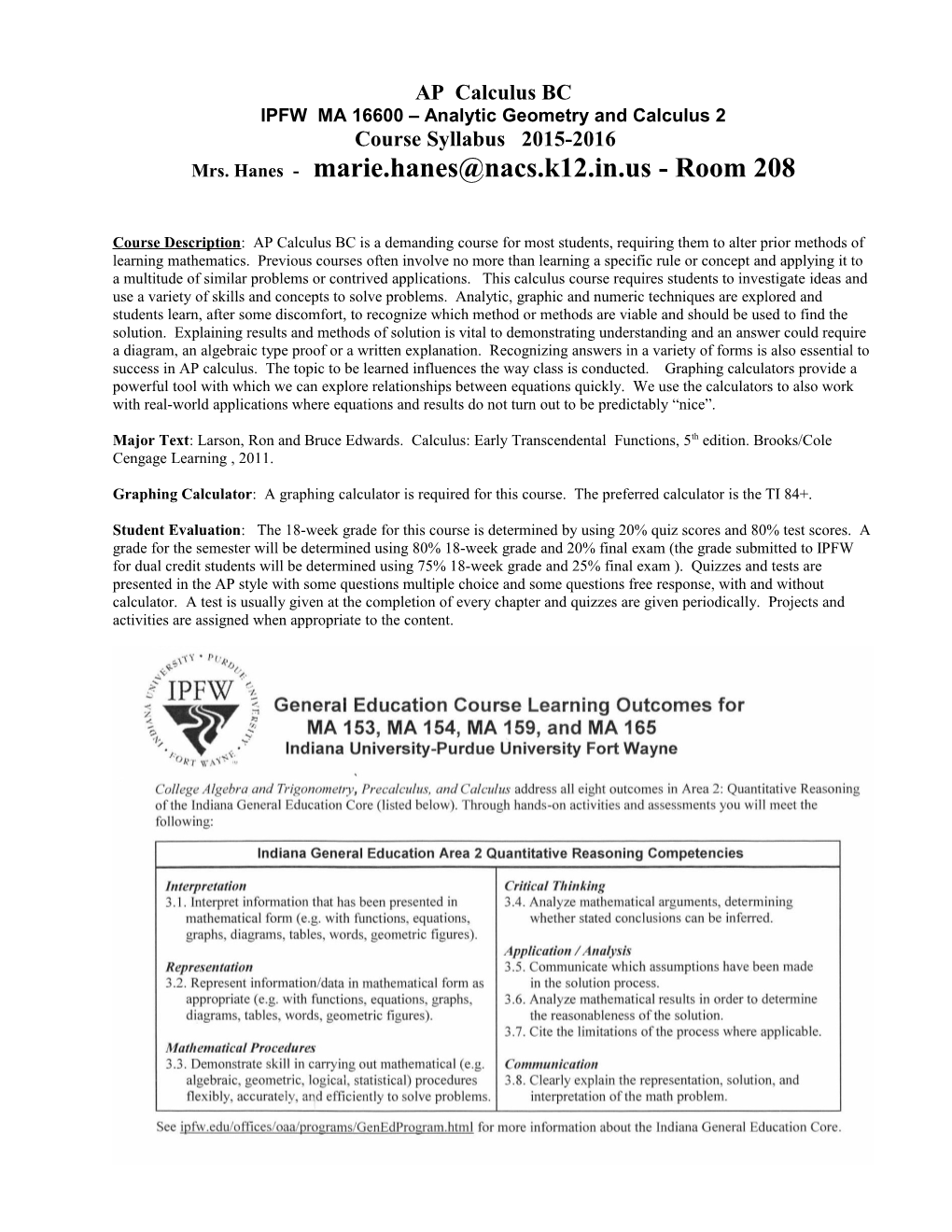# IPFW MA 16600 Analytic Geometry and Calculus 2AP CalculusBC

IPFW MA 16600 – Analytic Geometry and Calculus 2

Course Syllabus 2015-2016

Mrs. Hanes - - Room 208

Course Description: AP Calculus BC is a demanding course for most students, requiring them to alter prior methods of learning mathematics. Previous courses often involve no more than learning a specific rule or concept and applying it to a multitude of similar problems or contrived applications. This calculus course requires students to investigate ideas and use a variety of skills and concepts to solve problems. Analytic, graphic and numeric techniques are explored and students learn, after some discomfort, to recognize which method or methods are viable and should be used to find the solution. Explaining results and methods of solution is vital to demonstrating understanding and an answer could require a diagram, an algebraic type proof or a written explanation. Recognizing answers in a variety of forms is also essential to success in AP calculus. The topic to be learned influences the way class is conducted. Graphing calculators provide a powerful tool with which we can explore relationships between equations quickly. We use the calculators to also work with real-world applications where equations and results do not turn out to be predictably “nice”.

Major Text: Larson, Ron and Bruce Edwards. Calculus: Early Transcendental Functions, 5th edition. Brooks/Cole Cengage Learning , 2011.

Graphing Calculator: A graphing calculator is required for this course. The preferred calculator is the TI 84+.

Student Evaluation: The 18-week grade for this course is determined by using 20% quiz scores and 80% test scores. A grade for the semester will be determined using 80% 18-week grade and 20% final exam (the grade submitted to IPFW for dual credit students will be determined using 75% 18-week grade and 25% final exam ). Quizzes and tests are presented in the AP style with some questions multiple choice and some questions free response, with and without calculator. A test is usually given at the completion of every chapter and quizzes are given periodically. Projects and activities are assigned when appropriate to the content.Course Outline: The following course outline describes in general terms the variety of topics taught and their sequence. This course is designed to meet the expectations as set forth by the CollegeBoard AP for CalculusAB. Students will be expected to apply learned concepts to different types of problems and use various methods to describe or explain solutions. Solutions will be given in a variety of forms including algebraically, graphically and/or verbally (in oral or written form).

Limits and Derivatives (Chapter 2)

-Finding Limits Graphically and Numerically

-Evaluating Limits Analytically

-Continuity and One-Sided Limits

-Infinite Limits

Differentiation Rules (Chapter 3)

-Basic Differentiation Rules and Rates of Change

-Product and Quotient Rules and Higher-Order Derivatives

-Chain Rule

-Implicit Differentiation

-Derivatives of Inverse Functions

-Related Rates

Integration (Chapter 5)

-Antiderivaties and Indefinite Integration

-AreaReimann Sums

-Fundamental Theorem of Calculus

-Integration by Substitution

-Natural Logarithmic Function: Integration

Infinite Series (Chapter 9)

-Sequences

-Series and Convergence

-Integral Test and p-Series

-Comparisons of Series

-Alternating Series

-Ratio and Root Tests

-Taylor Polynomials and Approximations

-Power Series

-Taylor and Maclaurin Series

Differential Equations (Chapter 6)

-Slope Fields

-Growth and Decay

-Seperation of Variables

Techniques of Integration (Chapter 8)

-Basic Integration Rules

-Integration by Parts

-Integration by Partial Fractions

-Indeterminate Forms and L’Hopital’s Rule

-Improper Integrals

(Approximate end of first semester)

Conics, Parametric Equations and Polar Coordinates (Chapter 10)

-Conics

-Plane curves and parametric equations

-Polar coordinates

-Area and arc length in polar coordinates

Vector-Valued Functions (Chapter 12)

-Vectors

-Differentiation and Integration of vector functions

-Velocity and acceleration

Applications of Differentiation (Chapter 4)

-Extrema on an Interval

-Rolle’s Theorem and Mean Value

-Increasing/Decreasing Functions

-Concavity

-Curve Sketching

-Optimization

Applications of Integrals (Chapter 7)

-Areas between curves

-Volumes using disk, shell methods and with known cross sections Average value of a function

-Arc Length

Student Evaluation:

## Student grades will be figured with the following items

weighted by the percentages indicated below. The grade is

cumulativeover the 18 week semester.

Tests:80%

Quizzes 20%

Homework: 0%

Homework, when collected, must be turned in and complete.

An incomplete assignment will result in the student spending time

with me before and/or after school until the assignment is finished.

End of Semester Final Exam: 20% of semester

Dual credit students only: 25% of semester (for the grade submitted to IPFW)

Absent for a Test or Quiz: When a student is absent the day a test or quiz is given, the student is expected to take the test or quiz immediately upon their return. If many days were missed, discuss with me when the test will be taken. It is best if the test can be made up during study hall or before/after school.

Absent/Late Work Policy: Students are responsible for gathering missed work on their first day back to school whether they have class or not (block days). Any quiz or test taken on the day of absence should be made up immediately upon return. If multiple days were missed, discuss timing of make-up tests with me.

Class Expectations: 1. In the room when the bell rings.

1. Actively take part in all discussions, examples and notes.
2. Keep track of grades, check it on-line often.
3. Have appropriate supplies daily

textbook, paper, pencil , Graphing Calculator

Online Resources: CalcChat.com (solutions to odd numbered book problems)